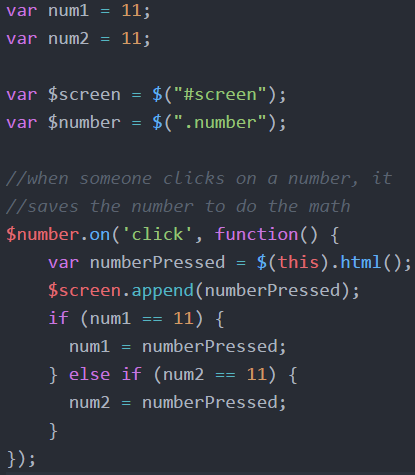# If – Calculator Project

If…a powerful question in life and in coding. Today we’ll be asking if a lot. We’re going to say, if the operator is plus, then add. If the operator is minus, then subtract. And so on. It looks like this.Please note: * is the symbol to tell the computer to multiply. Also parseInt(num1), is taking the html that was assigned to num1 and turning it into an integer, into a number. I talk about that in the video.

A simple if statement, says if (then the thing we are asking goes in the parentheses). If it’s true, then it will do whatever is in the curly brackets. If it’s not true, it will just move on to the next thing, whatever is after the closing curly bracket.

if (op == “+”) { answer = num1 + num2};

This says, if the operator is plus, then add the two numbers together to get the answer.

The double equals sign is how we compare numbers. You can use a triple equals sign to make sure they are really, really the same value and type of thing. The single equals sign assigns value. We use the single equals sign after answer because we want the number on the right assigned to the variable (answer) on the left.

So, how did I get the values of num1 and num2? With more if statements!This has a fancy if statement. You can say if (this) {do this} else {do that}.

This does even more. My code says if (this) {do this} else if (that) {do that}; otherwise, it just won’t do anything and will move on.

So what are these ifs asking?

There are other ways to solve this problem, but this was my solution. When a number is pressed, I want it to get saved to we can use it in our math problem. I can’t just save every number as num1, though. I need to save the first number as num1 and then the next number as num2.

So I use if. If num1 hasn’t been assigned a number yet, then make it equal to whatever number was pressed. If num1 has a number, then if num2 hasn’t been assigned a number, make num2 equal to whatever number was pressed. Those are the if and else if statements in writing.

Many would use something called Boolean values to check if a number had been assigned. Instead of num1 and num2 being equal to 11, I could make them equal to false, as in they have not been assigned a number. Then I could make them equal to true when they were assigned a number. I don’t like using true/false like that. I’m just telling you because that’s how others would do it. I often do something like assign a variable the value of 0 and then change it to 1. That’s the same exact thing as using true/false, but my brain just likes it better.

Here I assigned num1 and num2 the value of 11. I knew no one would be pushing number 11 on the number key. So if num1 or num2 equals 11, we know that no number was assigned to it yet. Then after my operation is complete, I set them back to 11 to start over.

Maybe you already see the new problem this will create. Now I can only add one digit numbers! We’ll work on that, next time.

You can see this lesson as a video.